# Calculus 3 : Stokes' Theorem

## Example Questions

1 2 3 4 5 6 7 9 Next →

### Example Question #81 : Surface Integrals

Let S be a known surface with a boundary curve, C.

Considering the integral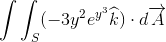, utilize Stokes' Theorem to determine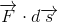for an equivalent integral of the form: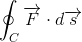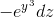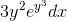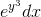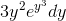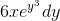Explanation:

In order to utilize Stokes' theorem, note its form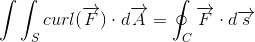The curl of a vector function F over an oriented surface S is equivalent to the function itself integrated over the boundary curve, C, of S.

Note that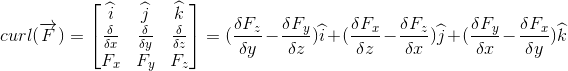From what we're told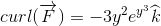And it can be inferred from this that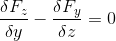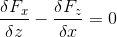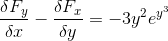A helpful approach can be to look at the right sides of the equations and see what variables are represented compared to what variables a vector component of F is being derived for. Doing this and integrating, we can infer that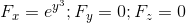and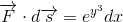### Example Question #81 : Stokes' Theorem

Let S be a known surface with a boundary curve, C.

Considering the integral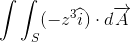, utilize Stokes' Theorem to determinefor an equivalent integral of the form: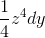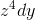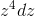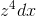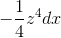Explanation:

In order to utilize Stokes' theorem, note its formThe curl of a vector function F over an oriented surface S is equivalent to the function itself integrated over the boundary curve, C, of S.

Note thatFrom what we're told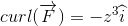And it can be inferred from this that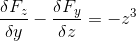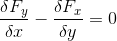A helpful approach can be to look at the right sides of the equations and see what variables are represented compared to what variables a vector component of F is being derived for. Doing this and integrating, we can infer that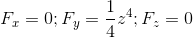and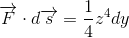### Example Question #81 : Surface Integrals

Let S be a known surface with a boundary curve, C.

Considering the integral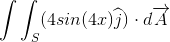, utilize Stokes' Theorem to determinefor an equivalent integral of the form: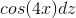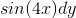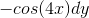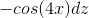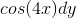Explanation:

In order to utilize Stokes' theorem, note its formThe curl of a vector function F over an oriented surface S is equivalent to the function itself integrated over the boundary curve, C, of S.

Note thatFrom what we're told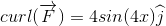And it can be inferred from this that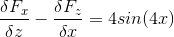A helpful approach can be to look at the right sides of the equations and see what variables are represented compared to what variables a vector component of F is being derived for. Doing this and integrating, we can infer that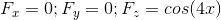and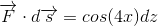### Example Question #84 : Stokes' Theorem

Let S be a known surface with a boundary curve, C.

Considering the integral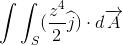, utilize Stokes' Theorem to determinefor an equivalent integral of the form: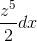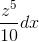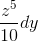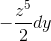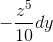Explanation:

In order to utilize Stokes' theorem, note its formThe curl of a vector function F over an oriented surface S is equivalent to the function itself integrated over the boundary curve, C, of S.

Note thatFrom what we're told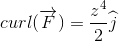And it can be inferred from this that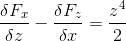A helpful approach can be to look at the right sides of the equations and see what variables are represented compared to what variables a vector component of F is being derived for. Doing this and integrating, we can infer that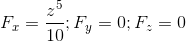and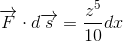1 2 3 4 5 6 7 9 Next →

### All Calculus 3 Resources